# NCERT Area Related to Circle Class 10 Chapter 12 Exercise – 12.2 Solution

## Exercise – 12.2

Q1. Find the area of a sector of a circle with radius 6 cm  if angle of the sector is 60º.

Solution: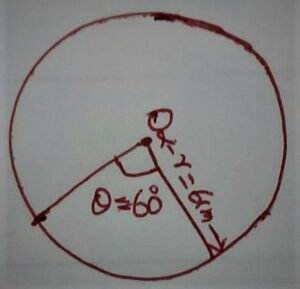θ = 60°

Area of Minor Sector = (θ /360) × π r²

⇒  Area of Minor Sector = (60/360) × 22/7 × (6)²

⇒ Area of Minor Sector = (60×22×6×6)/360×7

⇒ Area of Minor Sector = (22×6)/7

⇒ Area of Minor Sector = 132/7 cm²

Q.2. Find the area of Quadrant of a Circle whose Circumference = 22 cm.

Solution:

Circumference of Circle = 22 cm

2 πr = 22

⇒ 2 × 22/7 × r = 22

⇒  r = (22×7)/(2×22)

⇒ r = 7/2 cm

Area of Quadrant = 1/4 × πr²

⇒ Area of Quadrant = 1/4 × πr²

⇒ Area of Quadrant = 1/4 × 22/7 × 7/2 × 7/2

⇒ Area of Quadrant = 11×7 / 4×2

⇒ Area of Quadrant = 77/8 cm²

Q.3. The length of  the minute hand of a clock is 14 cm. Find the area swept by the minutes hand in 5 minutes.

Given:

Length of minute hand of a clock = 14 cm.

To Find:

Area swept by the minute hand in 5 minutes = ?

Solution:

In 1 hour = 60 minutes angle swept by minute hand = 360º

∴ In 1 minutes Angle swept by minute hand = 360º/60º =6º

∴ In 5 minutes Angle swept by minute hand = 6º × 5 = 30º

Area swept by the minute hand in 5 minutes = (θ/360°)× πr²

⇒ Area swept by the minute hand in 5 minutes = (60º/360°)× 22/7 × 14²

⇒ Area swept by the minute hand in 5 minutes = (60º/360°)× 22/7 × 14 × 14

⇒ Area swept by the minute hand in 5 minutes = (1/6°)× 22 × 2 × 14 = 154/3 cm²

Q.4. A Chord of a circle of radius 10 cm subtends a right angle at the center. Find the area of the corresponding :

(i) Minor Segment .

(ii) Major Sector . (use π = 3.14)

Solution: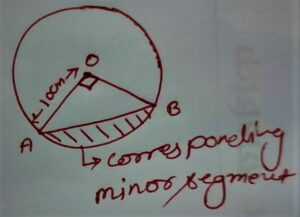r = 10 cm

θ = 90º

(i)  Area of corresponding minor segment = [(θπ/360°) – sin(θ/2)cos(θ/2) ] r²

⇒ Area of corresponding minor segment = [(90°π/360°) – sin(90°/2)cos(90°/2) ] 10²

⇒ Area of corresponding minor segment = [ (3.14/4) – sin45ºcos45º ] 10²

⇒ Area of corresponding minor segment = [ (3.14/4) – 1/√2×1/√2 ] 10²

⇒ Area of corresponding minor segment = [ (157/200) – 1/√2×1/√2 ] 10²

⇒ Area of corresponding minor segment = [ (157/200) – 1/2 ] 10²

⇒ Area of corresponding minor segment = [ (157/200) – 1/2 ] 100

⇒ Area of corresponding minor segment = [ (157/200) – 1/2 ] 100

⇒ Area of corresponding minor segment = [ (157-100)/200 ] 100

⇒ Area of corresponding minor segment = [ 57/200 ] 100

⇒ Area of corresponding minor segment =  57/2

⇒ Area of corresponding minor segment =  28.5 cm²

(ii) Area of Major Sector = (360-θ)πr²/360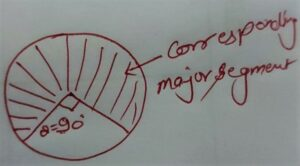⇒  Area of Major Sector = (360-90)×3.14×10²/360

⇒  Area of Major Sector = 471/2

⇒  Area of Major Sector = 471/2

⇒  Area of Major Sector = 235.5 cm²

Q.5. In a Circle of radius 21 cm, an arc subtends an angle of 60º at the center . Find:

(i) The length of the arc

(ii) Area of the Sector formed by the Arc

(iii) Area of the Segment Formed by the Corresponding chord

Solution: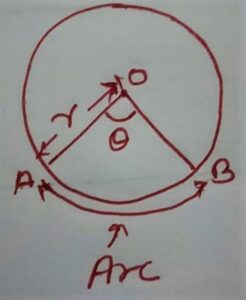r = 21 cm

θ = 60°

(i) The Length of the Arc = (θ × 2πr)/360

⇒ The Length of the Arc = (θ × 2πr)/360

⇒ The Length of the Arc = (60×2×22×21)/7×360

⇒ The Length of the Arc = 22 cm.

(ii) Area of Sector Formed by the Arc = θπr²/360

⇒ Area of Sector Formed by the Arc = 60×22×21²/7×360

⇒ Area of Sector Formed by the Arc = 60×22×21²/7×360

⇒ Area of Sector Formed by the Arc = 231 cm²

(iii) Area of Segment Formed by the Corresponding Chord = [(θπ/360°) – sin(θ/2)cos(θ/2) ] r²

⇒ Area of Segment Formed by the Corresponding Chord = [60º/360º × 22/7 – sin(60/2)cos( 60/2) ] 21²

⇒ Area of Segment Formed by the Corresponding Chord = [(1/6) × 22/7 – sin30cos30]×21×21

⇒ Area of Segment Formed by the Corresponding Chord = [(1/6) × 22/7 – 1/2×√3/2]×21×21

⇒ Area of Segment Formed by the Corresponding Chord = [ 11/21 – √3/4 ] × 21 × 21

⇒ Area of Segment Formed by the Corresponding Chord = [(44 – 21√3)/21×4]×21×21

⇒ Area of Segment Formed by the Corresponding Chord = [(44 – 21√3)/21×4]×21×21

⇒ Area of Segment Formed by the Corresponding Chord = [(44 – 21√3)/21×4]×21×21

⇒ Area of Segment Formed by the Corresponding Chord = [(44 – 21√3)/4]×21

⇒ Area of Segment Formed by the Corresponding Chord = [(44 – 21√3)/4]×21

⇒ Area of Segment Formed by the Corresponding Chord = [(44 – 21√3)/4]×21

⇒ Area of Segment Formed by the Corresponding Chord = [(231 – 441√3)/4]cm²

Q.6. A chord of a circle of radius 15 cm subtends an angle of 60º at the center. Find the areas of  the corresponding minor and major segments of the circle, [ use π = 3.14 ]

Given:

C (o ,r)

r = 15 cm

θ = 60°

To Find:

(i) Area of corresponding minor segment =?

(ii) Area of corresponding major segment =?

Solution:

Area of corresponding minor segment = [(θπ/360°) – sin(θ/2)cos(θ/2) ] r²

⇒ Area of corresponding minor segment = [(60ºπ/360°) – sin(60º/2)cos(60º/2) ] 15²

⇒ Area of corresponding minor segment = [3.14/6  – sin30ºcos30º ] 15×15

⇒ Area of corresponding minor segment = [157/3×100 – 1/2 × √3/2 ] 15×15

⇒ Area of corresponding minor segment = [ 157/300 – 1/2 × √3/2 ] 15×15

⇒ Area of corresponding minor segment = [157/300 – 1.73/4] 15×15

⇒ Area of corresponding minor segment = [(157×4 – 1.73×300)/300×4] 15×15

⇒ Area of corresponding minor segment = [(628 – 519)/300×4] 15×15

⇒ Area of corresponding minor segment = [(628 – 519)/300×4] 15×15

⇒ Area of corresponding minor segment = [(109)/300×4] 15×15

⇒ Area of corresponding minor segment = 327/16

⇒ Area of corresponding minor segment = 20.4375 cm²

(i) Area of major segment = Area of the circle – Area of the minor segment

⇒ Area of major segment = πr² – 20.4375 cm²

⇒ Area of major segment = 3.14×15×15 – 20.4375 cm²

⇒ Area of major segment = 3.14×225 – 20.4375 cm²

⇒ Area of major segment = 3.14×225 – 20.4375 cm²

⇒ Area of major segment = 706.5 – 20.4375 cm²

⇒ Area of major segment = 686.0625 cm²

Q.7. A chord of a circle of radius 12 cm subtends an angle of 120º at the center. Find the area of the corresponding segment of the circle [ use π = 3.14, √3 = 1.73 ]

Solution: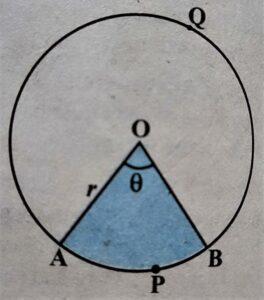Radius = r = 12 cm

θ = 120°

Area of corresponding segment = [(θπ/360°) – sin(θ/2)cos(θ/2) ] r²

⇒ Area of corresponding segment = [(120×3.14/360°) – sin(120/2)cos(120/2) ] 12²

⇒ Area of corresponding segment = [(3.14/3) – sin(60)cos(60) ] 12²

⇒ Area of corresponding segment = [(3.14/3) – √3/2 × 1/2 ] 12²

⇒ Area of corresponding segment = [(3.14/3) – √3/2 × 1/2 ] 12²

⇒ Area of corresponding segment = [(3.14/3) – √3/4] 12²

⇒ Area of corresponding segment = [(12.56 – 3√3)12] 12²

⇒ Area of corresponding segment = [(12.56 – 3×1.73)12] 12²

⇒ Area of corresponding segment = (7.37/12)12×12

⇒ Area of corresponding segment = 7.37 × 12

⇒ Area of corresponding segment = 88.44 cm²

Q.8. A horse is tied to peg at one corner of a square shaped grass field of side 15 m by means if a 5 m long rope (see in fig. 12.11) Find: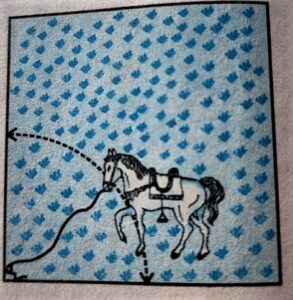(i) The area of that part of the field in which the horse can graze

(ii) The increase in the grazing area if the rope were 10 m long instead of 5 m.[use π = 3.14 ]

Solution: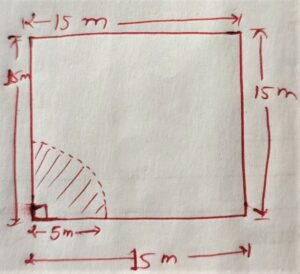Given that the grass field is a square shape

∴ θ = 90º

Length of Rope = Radius = r = 5 cm

The area of that part of the field in which the horse can graze = Area of sector

⇒ The area of that part of the field in which the horse can graze = (θ/360º)×πr²

⇒ The area of that part of the field in which the horse can graze = (90º/360º)×3.14×5²

⇒ The area of that part of the field in which the horse can graze = (157 × 5 × 5)/2×100

⇒ The area of that part of the field in which the horse can graze = 157/8

⇒ The area of that part of the field in which the horse can graze = 19.625 m²

(ii) The increase in the grazing area if the rope were 10m long instead of 5 m = Area of the sector with r=10 m –                                                                                                                                                             Area of the sector with r= 5 m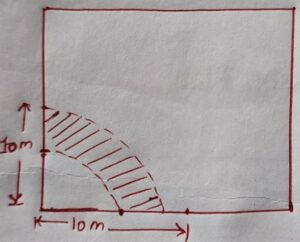⇒ The increase in the grazing area if the rope were 10m long instead of 5 m = (θ/360)×πr² – 157/8

⇒ The increase in the grazing area if the rope were 10m long instead of 5 m = (90/360)×3.14×10×10 – 157/8

⇒ The increase in the grazing area if the rope were 10m long instead of 5 m = 157/2 – 157/8

⇒ The increase in the grazing area if the rope were 10m long instead of 5 m = (628 – 157)/8

⇒ The increase in the grazing area if the rope were 10m long instead of 5 m = 471/8

⇒ The increase in the grazing area if the rope were 10m long instead of 5 m = 58.875 m²

Q.9. A brooch is made with silver wire in the form of a circle with a diameter 35 mm. The wire is also used in making diameters which divide the circle into 10 equal sectors as shown in fig. 12.12 find: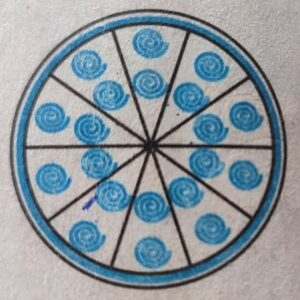(i) The total length of the silver wire required =?

(ii) The area of each sector of the brooch =?

Solution:

Diameter of circle = 35 mm

Total number of diameters used = 5

Number of sector = 10

(i) The total length of the silver wire required = 5 × Diameter + Circumference

⇒ The total length of the silver wire required = 5 × 35 + 2πr

⇒ The total length of the silver wire required = 5 × 35 + 2×22/7×35/2

⇒ The total length of the silver wire required = 175 + 22 × 5

⇒ The total length of the silver wire required = 175 + 110

⇒ The total length of the silver wire required = 285 mm

(ii) The area of each sector of the brooch = (θ/360)πr²

Angle subtends between sector = 360/10=36º

⇒ The area of each sector of the brooch = (36/360) × 22/7 × 35/2 × 35/2

⇒ The area of each sector of the brooch = (11×35)/4

⇒ The area of each sector of the brooch = 385/4 mm²

Q.10. An umbrella has 8 ribs which are equally spaced (see fig. 12.13), Assuming the umbrella to be a flat circle of radius 45 cm, Find the area between the two consecutive ribs of the umbrella.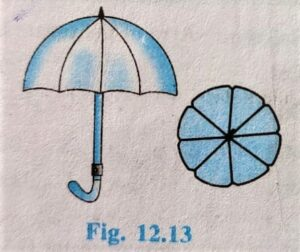Solution:

In Umbrella :

Numbers of ribs = 8

Area between two consecutive ribs = 360/8 = 45º

Area between two consecutive ribs = Area of sector

Area between two consecutive ribs = (θ/360)×πr²

⇒ Area between two consecutive ribs = (45/360)×(22/7)×45²

⇒ Area between two consecutive ribs = (45/360)×(22/7)×45×45

⇒ Area between two consecutive ribs = (11×45×45)/7×4

⇒ Area between two consecutive ribs = 22275/28 cm²

Q.11. A car has two wipers which do not overlap. Each wiper has a blade of length 25 cm sweeping through an angle of 115°. Find the total area cleaned at each sweep of the blades.

Solution:

Length of Blade of each wiper =25 cm.

θ = 115°

Total area cleaned by each wiper = Area of sector

⇒ Total area cleaned by each wiper = (θ/360º)×πr²

⇒ Total area cleaned by each wiper = (115/360º)×22/7×25²

⇒ Total area cleaned by each wiper = 115×11×5×25/36×7

⇒ Total area cleaned by each wiper = 158125/36×7

Now total area cleaned at each sweep of the blades = 2×Area cleaned by each wiper

⇒ Total area cleaned at each sweep of the blades = 2× 158125/36×7

⇒ Total area cleaned at each sweep of the blades = 158125/126 cm²

Q.12. To warn ships for underwater rocks, a lighthouse spreads a red-colored light over a sector of angle 80° to a distance of 16.5 km. Find the area of the sea over which the ships are warned.[use π = 3.14]

Solution:

θ = 80°

r = 16.5 km

The area of the sea over which the ships are warned = (θ/360º)×πr²

⇒ The area of the sea over which the ships are warned = (80/360º)×3.14×16.5×16.5

⇒ The area of the sea over which the ships are warned = (314×11×165)/3×100×10

⇒ The area of the sea over which the ships are warned = 3454×55/1000

⇒ The area of the sea over which the ships are warned = 189970/1000

⇒ The area of the sea over which the ships are warned = 189.97 km²

Q.13. A round table cover has six equal designs as shown in fig. 12.14. If the radius of the cover is 28 cm, find the cost of the radius of the cover is 28 cm, find the cost of making the designs at the rate of RS 0.35 per cm²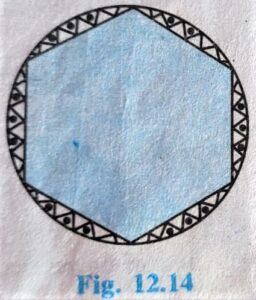[use √3 = 1.7]

Solution:

Radius of the cover = 28 cm,

The cost of designs at the rate of Rs 0.35/cm²

Number of Designs = 6

θ = 60°

Each Design have = 60° with Center

Total Area if design = 6×Area segment

⇒ Total Area of Design = 6×Area segment

⇒ Total Area of Design = 6×[(θπ/360°) – sin(θ/2)cos(θ/2) ] r²

⇒ Total Area of Design = 6×[(60°×22/7×360°) – sin(60/2)cos(60/2) ]×28²

⇒ Total Area of Design = 6×[(60°×22/7×360°) – sin(30°)cos(30°) ]×28²

⇒ Total Area of Design = 6×[ 11/21 – 1/2 × √3/2 ]×28×28

⇒ Total Area of Design = 6×[(11×4 – 21√3)/21×4  ]×28×28

⇒ Total Area of Design = 6×[(44 – 21√3)/21×4  ]×28×28

⇒ Total Area of Design = 6×[(44 – 21×1.7)/21×4]×28×28

⇒ Total Area of Design = (6×8.3×28×28)/21×4

⇒ Total Area of Design = 2× 8.4 × 28

⇒ Total Area of Design = 464.8 cm²

Hence the cost of making the designs at the rate of Rs 0.35 = 0.35×464.8 =162.68 Rs

Q.14. Take the correct answer in the following:

Area of a sector of angle P (in degrees)of a circle with radius R is :

(A) p/180 ×2πR

(B) p/180 ×πR²

(C) p/360 ×2πR

(D) p/720 ×2πR²

Ans (D)

Area of Sector = θ/360 × πR²

Area of Sector = p/360 × πR²

Area of Sector = p/720 × πR²